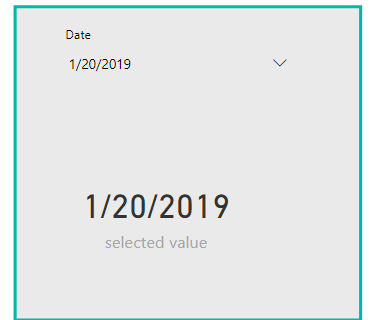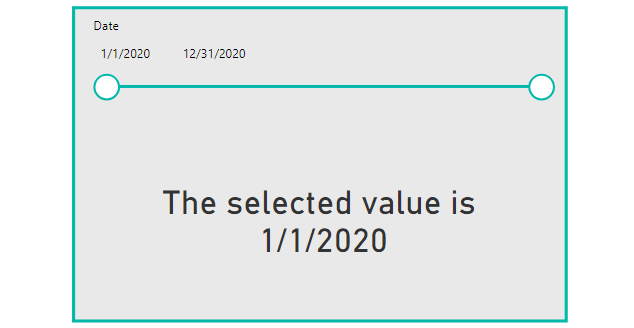# power bi measure based on slicer

0 like 0 dislike
5.8k views
in Power BI

In my power BI dashboard, I have a date slicer, and I want to pass and use slected slicer value in dax fomula in a measure.

This is the date slicer and I need when selecting a date I can get the selectd value and use it in measure fromula, so basically, How can I use slicer value in a measure in Power BI?1 like 0 dislike
by 64 137 299
selected by

## Use slicer value in a measure in Power BI

To get the slicer selected value and use it in a DAX formula in Measure, you can use one of the below options

### SELECTEDVALUE()

Use `SELECTEDVALUE()` to get the selected slicer value in your DAX formula in a Measure as below

``````selected value = SELECTEDVALUE('Calendar'[Date])
``````

This would be helpful for the list or dropdown date selection slicer.

Output### Min Approach

If you are using `between date slicer`, you also use the Min approach to pass the selected slicer value in a Measure as below

``````Slicer Selected Value = Var selectedValue = MIN('Calendar'[Date])
Return
CALCULATE(SUM('Calendar'[Date]),'Calendar'[Date] = selectedValue)
``````

Or this formula

``````Slicer Selected Value = CALCULATE(Min('Calendar'[Date]),ALLSELECTED('Calendar'))
``````

OutputNote: The above formula will return the minimum selected value of the slicer in a Power BI Measure where the `'Calendar'[Date]` is the column used in the slicer as shown below:by 18 23 29
0 0
by 18 23 29
0 0
and how can I use the slicer value in calculated column instead the measure in power bi
by 64 137 299
1 0
by 18 23 29
0 0
Thank you for your great help, I will add a new question now and let you know!
0 like 0 dislike
by 2 10 14

Yes, In your case, you can simply use MAX or MIN funtion, so it will take the selected value. Also you can use SELECTEDVALUE.

Yet, in general to be able to have different measures based on the slicer, you need to nest them in switch.

You can create a parameter table hosting the options with datatable, no need for relationship with other tables.
Have your measures ready for each option and then use SWITCH and SELECTEDVALUE together and nest your measures inside.

This video explains it in two ways thoroughly